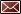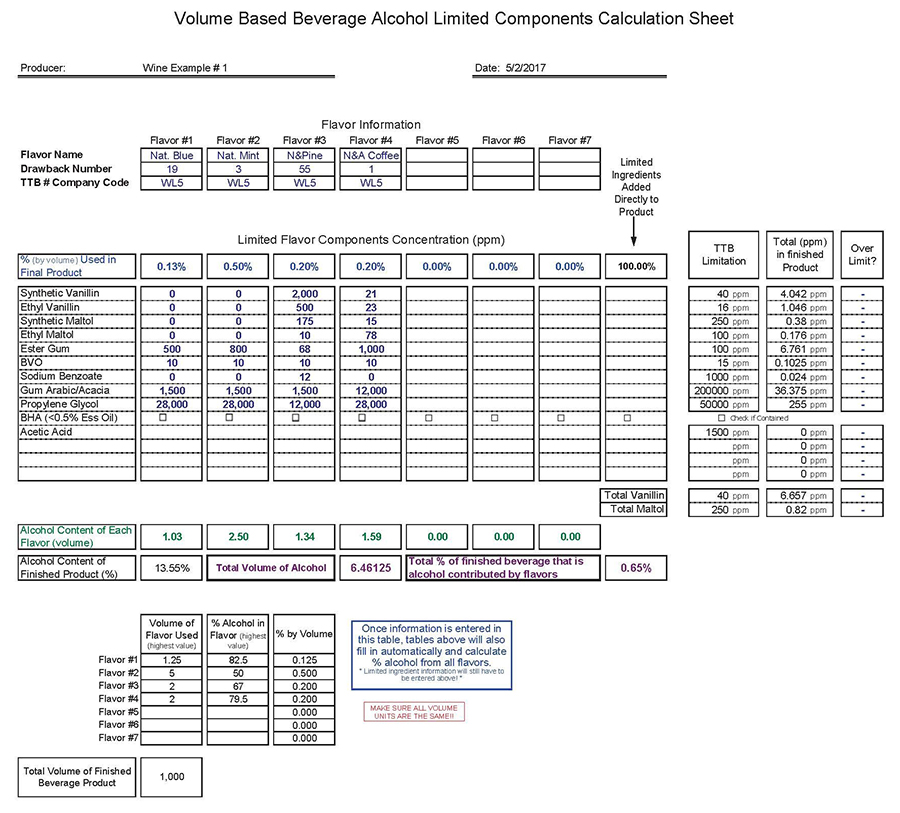Get Email Updates Back to Nonbeverage Products Laboratory page

# Drawback Tutorial

Example formulas

Simple Mixture 1

In this example, items 9 and 10 are relatively easy to calculate because all of the ethanol is coming from one source (see the first line in item# 13) and the yield is 100% (simple mixture).Step 1. Start with the ethanol: 7.0 pounds have been added to the mixture.  To convert pounds to gallons, 7.0 pounds is divided by the density (in pounds per gallon) to give the volume: 7.0 ÷ 6.7943 = 1.03 gallons again, this is: pounds ÷ (pounds per gallon) = gallons Always be careful about units (do not mix metric and English units.) Step 2. Once the value in terms of volume has been calculated, you need to compensate for the fact that the ethanol is only 95% pure (the other 5% is water). You must multiply the volume you calculated in (1) by the percent ethanol to get the volume of absolute ethanol: 1.03 gallons × 0.95 = 0.979 gallons of absolute ethanol Click on the image to enlarge

Step 3. To calculate item 9, you would divide 0.979 gallons of absolute ethanol by the yield (9.5 gallons):

0.979 gallons ÷ 9.5 gallons = 0.103 or 10.3%

For this formula, items 9 and 10 are calculated the same way. The tolerance is applied only to item 10 as described in the tolerance table for a simple mixture.